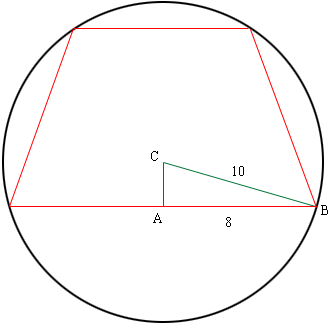Quandaries and Queries My problem is as follows: An isosceles trapezoid with bases of lengths 12 and 16 is inscribed in a circle with a radius of 10.  The center of the circle lies in the interior of the trapezoid.  Find the area of the trapezoid. Thanks for your help! Bruce I am a student-teacher Grade level 9th to 12th Hi Bruce, The area of a traposoid is the average of the lengths of the parallel sides times the distance between the parallel lines. I drew a rough sketch of your situation.C is the center of the circle and triangle ABC is a right triangle so you can use Pythagoras' theorem to find |AC|. Can you complete the problem now? Penny Go to Math Central